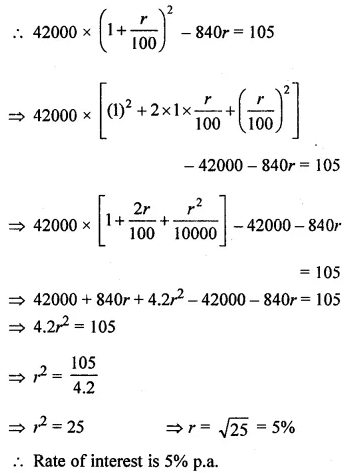# ML Aggarwal Class 8 Solutions for ICSE Maths Chapter 8 Simple and Compound Interest Objective Type Questions

## ML Aggarwal Class 8 Solutions for ICSE Maths Chapter 8 Simple and Compound Interest Objective Type Questions

Mental Maths
Question 1.
Fill in the blanks:
(i) The money borrowed (lent or invested) is called …………
(ii) the additional money paid by the borrower to the moneylender in lieu of the money used is called …………
(iii) In simple interest, the principal ………… for the whole loan period.
(iv) In compound interest the ………… goes on changing every conversion period.
(v) The time after which the interest is added each time to form a new principal is called …………
(vi) If the interest is compounded semi-annually then semi-annually rate is ………… of the annual rate.
Solution: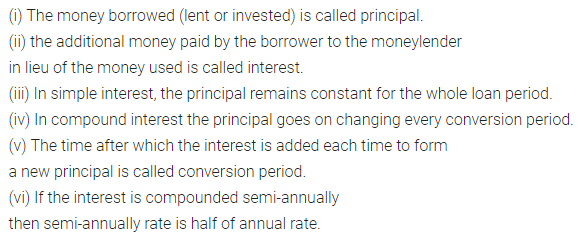Question 2.
State whether the following statements are true (T) or false (F):
(i) The interest paid by the banks, post offices, insurance companies is simple interest.
(ii) Compound interest is calculated on the amount of the previous year.
(iii) In compound interest, the principal remains constant for the whole period.
(iv) The time from one specified interest period to the next period is called the conversion period.
(v) If the interest is compounded quarterly then there are 2 conversion periods in a year.
Solution: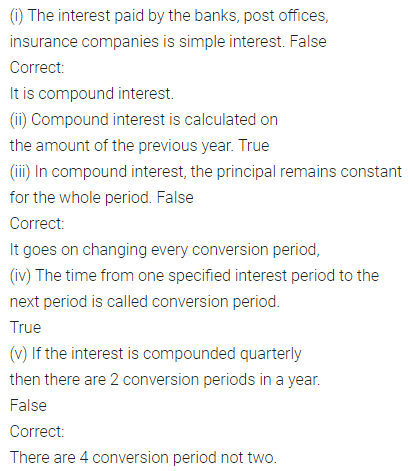Multiple Choice Questions
Choose the correct answer from the given four options (3 to 9):
Question 3.
The compound interest on ^1000 at 10% p.a. for 2 years is
(a) ₹190
(b) ₹210
(c) ₹1210
(d) ₹200
Solution: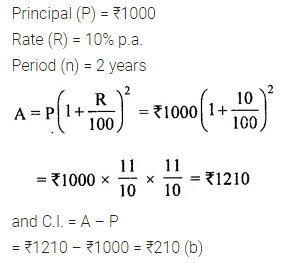Question 4.
The compound interest on ₹5000 at 20% per annum for $$1 \frac{1}{2}$$ years compounded half yearly is
(a) ₹6655
(b) ₹1655
(c) ₹50
(d) ₹1000
Solution: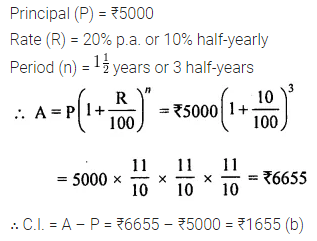Question 5.
The compound interest on ₹10000 at 8% per annum for 6 months compounded quarterly is
a) ₹408
(b) ₹10404
(c) ₹404
(d) ₹400
Solution:Question 6.
The time periods and rate for a sum taken at 8% p.a. for $$1 \frac{1}{2}$$ years compounded half yearly are
(a) n = 3, R = 4%
(b) n = 6, R = 2%
(c) n = 3, R = 2%
(d) n = 6, R = 4%
Solution: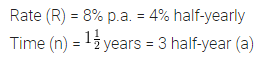Question 7.
If ₹12000 taken for 2 years at 4% per annum compounded quarterly, then time period and rate is
(a) n = 2, R = 16%
(b) n = 4, R = 1 %
(c) n = 8, R = 1%
(d) n = 8, R = 16%
Solution: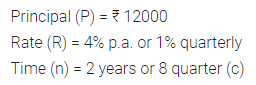Question 8.
If the number of conversion periods ≥ 2, then compound interest is
(a) less than or equal to the simple interest
(b) greater than or equal to the simple interest
(c) less than simple interest
(d) greater than simple interest
Solution:Question 9.
The time in which ₹6000 amounts to ₹7986 at 10% p.a. compounded annually is
(a) 2 years
(b) 3 years
(c) 4 years
(d) 5 years
Solution:Value Based Question
Question 1.
A person wants to invest ₹ 100000 in fixed deposit scheme for 2 years. His financial advisor explained to him two types of schemes first is yielding 10% p.a. compounded annually, second is yielding 10% p.a. compounded semi-annually. Which scheme is better and why? Why investment is important for future life?
Solution:Higher Order Thinking Skills (Hots)
Question 1.
A certain sum of money is invested at the rate of 5% per annum compound interest, the interest compounded annually. If the difference between the interests of the third year and the first year is ₹102.50. Find the sum.
Solution: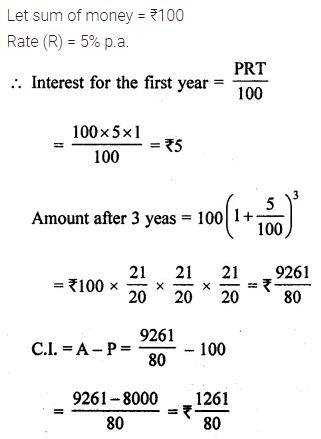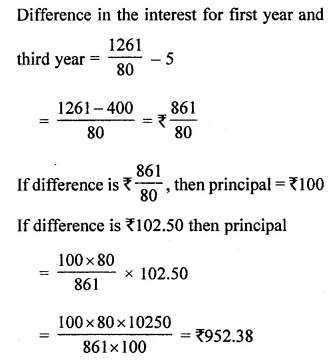Question 2.
The difference between the compound interest and the simple interest on ₹42000 for two years is ₹105 at the same rate of interest per annum. Find
(i) the rate of interest
(ii) the compound interest earned in the second year.
Solution: